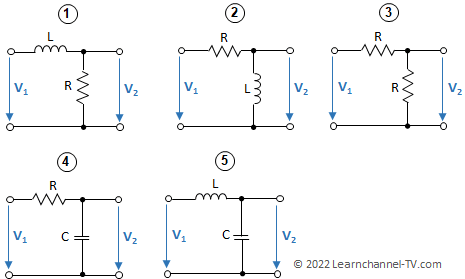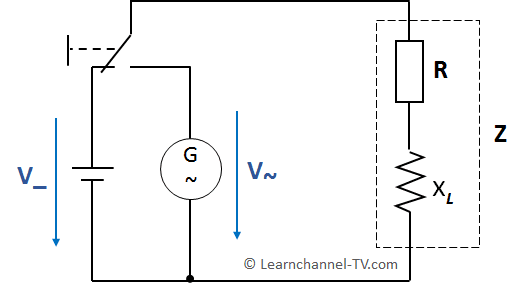### Exercises - Inductivity at AC voltage

Inductance connected to alternating voltage – exercises

A relay labeled V~ = 12 V is accidentally connected to 12 V DC. What will happen?

In which circuit does the output voltage V2 increase as the frequency of the sinusoidal input voltage V1 increases?Inductance connected to alternating voltage – exercises

How can you measure the impedance of a coil?

Exercise 1

An ideal coil with L = 35 mH is connected to an AC voltage with a frequency of f = 50 Hz. How big is the inductive reactance XL?

Exercise 2

An ideal coil with a reactive resistance of 6.3 kΩ is connected to a frequency of 60 Hz. Determine the inductance of the coil.

Exercise 3

A real coil has an ohmic resistance (wire resistance) and a reactance. To determine these two resistances, the coil (see measuring circuit) is connected to a DC voltage of 30 V and in the following to AC voltage of 50 V / 50 Hz. The current is 2.5 A each.Real world Inductor - how to determine the ohmic resistance and the Impedance

Determine

- The ohmic resistance R and the inductive reactance Xbl at 50 Hz
- The inductance L
- The phase shift angle at AC voltage.